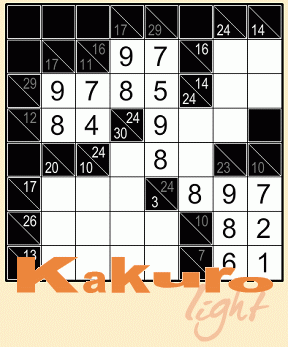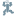# Kakuro Light

• Currently 4.3/5
• 1
• 2
• 3
• 4
• 5Mmm, crosswords! Mmm, sudoku! (*collision) Hey, you got crosswords in my sudoku! And you got sudoku in my crosswords! The mix of numbers and logic isn't that unlikely of a combination with Kakuro Light, the latest puzzle pack released by Conceptis in their Conceptis Light series. By putting numbers into the grid so that each row and column meet their given requirements, you can solve these puzzles of chocolatey, peanut buttery goodness.

Each puzzle starts out empty, save for the clue numbers in triangles at the top of each column and left-hand edge of each row. These clue numbers tell you the sum of all the digits in the row associated with that clue. You can use any numbers from 1 to 9 to make your sums, but remember the important sudoku rule that no digit can appear more than once in any given string of numbers that make a sum. (Note that a row or column may be broken by a black box. It's legal to have a digit appear in both of these separate strings of digits.) The numbers don't have to appear in any particular order, as long as all directions have their respective sums accounted for.

After a while, you'll spot certain patterns that make puzzle-solving much easier, like common sums (a 3 as the sum for a two-box string will always be 1-2 in some order, a 17-sum for the same length is always 8-9, etc.). While the smaller puzzles are perfect for beginners to get the hang of how to play, the difficulty ramps up nicely in the larger puzzles to keep you searching for the best next move. It only takes a bit of logic and some simple sum-searching to solve these Kakuro challenges!

Play Kakuro Light

### Walkthrough Guide

(Please allow page to fully load for spoiler tags to be functional.)

Kakuro Tips:

• One important tip for quickly solving Kakuro puzzles is to remember minimum and maximum values for strings of numbers of a certain length. For example, if a string is two digits long, and has a target sum of 3, you know the numbers in that string must be 1 and 2 in some order. Similarly, if the target sum is 4, the numbers must be 1 and 3 (2 and 2 would be illegal). On the other end of the spectrum, if a string of two numbers has a target sum of 17, you know it must contain a 9 and 8, while a sum of 16 must have 9 and 7. For a full list of sums you can automatically assume, click this spoiler tag:

For a two-box string:

• 3 = 1+2

• 4 = 1+3

• 17 = 9+8

• 16 = 9+7

For a three-box string:

• 6 = 1+2+3

• 7 = 1+2+4

• 24 = 7+8+9

• 23 = 6+8+9

For a four-box string:

• 10 = 1+2+3+4

• 11 = 1+2+3+5

• 30 = 6+7+8+9

• 29 = 5+7+8+9

For a five-box string (getting the hang of this yet?):

• 15 = 1+2+3+4+5

• 16 = 1+2+3+4+6

• 35 = 5+6+7+8+9

• 34 = 4+6+7+8+9

For a six-box string:

• 21 = 1+2+3+4+5+6

• 22 = 1+2+3+4+5+7

• 39 = 4+5+6+7+8+9

• 38 = 3+5+6+7+8+9

For a seven-box string:

• 28 = 1+2+3+4+5+6+7

• 29 = 1+2+3+4+5+6+8

• 42 = 3+4+5+6+7+8+9

• 41 = 2+4+5+6+7+8+9

For an eight-box string: Rather than typing out every combination, here's the trick to use here: Take a look at the target sum, and subtract it from 45 (which is the sum of all of the digits from 1 to 9). Whatever the difference is is the number to omit from your string. For example, if the sum is 43, your string is 1+3+4+5+6+7+8+9, omitting the 2.
For a nine-box string: USE ALL THE NUMBARS! (It's best if you imagine a stick-figure version of me waving a 9 in the air as I shout that.)

• Look for spots where any of the given patterns cross as starting points. For example, if you see two strings of two boxes with the sums of 16 and 17 intersect, you know the shared box has a 9.

• Even without using the above list of givens, you can also make good progress by looking for the intersections of high and low sums. For example, if you see two strings of two boxes with the sums 14 and 6 intersect, you know the shared box has a 5, because it's the highest possible digit for the sum of 6, and also the lowest possible digit for the sum of 14.

• Don't forget to eliminate possibilities! If you click the smaller shaded box in each square, you can write a small number in the box to remind you of what's possible in that square or not.

• Don't be afraid of good-old-fashioned trial and error! Again, use the tiny numbers to assist you on guesswork.

Kakuro Light Solutions

Levels 1 (8x8)

Puzzle 1:

Puzzle 2:

Puzzle 3:

Puzzle 4:

Puzzle 5:

Puzzle 6:

Puzzle 7:

Puzzle 8:

Puzzle 9:

Puzzle 10:

Level 2 (9x9)

Puzzle 1:

Puzzle 2:

Puzzle 3:

Puzzle 4:

Puzzle 5:

Puzzle 6:

Puzzle 7:

Puzzle 8:

Puzzle 9:

Puzzle 10:

Level 3 (10x10)

Puzzle 1:

Puzzle 2:

Puzzle 3:

Puzzle 4:

Puzzle 5:

Puzzle 6:

Puzzle 7:

Puzzle 8:

Puzzle 9:

Puzzle 10:

At last!

I've been waiting forever for conceptis to release a Kakuro edition of the conceptis lite seriesSeptember 15, 2011 10:03 AM

YESSSS

YESSSSSS

Finally a way to play Kakuro on my computer, without the aid of the Guardian's puzzle section and Paint !

There is only a limited amount, though. >:

Please consider creating a Casual Gameplay account if you're a regular visitor here, as it will allow us to create an even better experience for you. Sign-up here!
• You may use limited HTML tags for style:
(a href, b, br/, strong, em, ul, ol, li, code, spoiler)
HTML tags begin with a less-than sign: < and end with a greater-than sign: >. Always. No exceptions.
• To post spoilers, please use spoiler tags: <spoiler> example </spoiler>
If you need help understanding spoiler tags, read the spoiler help.
• No link dropping, no domains as names; do not spam, and do not advertise! (rel="nofollow" in use)

## Monkey Go Happy Roundup 66 - Retro

3,586 Views
chrpa Jayisgames needs your help to continue providing quality content. Click for details Welcome to the Roundup 66 - Retro with four games! After you find the ten monkeys in the chapter, look in the inventory. You will find a...  ...

## Monkey GO Happy Roundup 65

3,388 Views
chrpa Jayisgames needs your help to continue providing quality content. Click for details Welcome to the Roundup 65 with three games! As mentioned in the previous roundups, only odd-numbered episodes are featured since even-numbered are for Robin Vencel's patrons (the...  ...

## Weekday Escape N°376

7,820 Views
chrpa Jayisgames needs your help to continue providing quality content. Click for details Hi! Weekday Escape and Weekday Puzzle are here! First we have two new cans from tomoLaSiDo and then two small rooms from isotronic. That's all for this...  ...

## Mobile Monday N°340

6,365 Views
chrpa Jayisgames needs your help to continue providing quality content. Click for details Welcome to Mobile Monday! We have another beautiful game from Nicolet and it's a winter game as it should be. Tasuku Yahiro have released another of their...  ...

### HELP Jayisgames.com